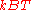xJoule per moleEncyclopedia
The joule per mole is an SI
Si
Si, si, or SI may refer to :- Measurement, mathematics and science :* International System of Units , the modern international standard version of the metric system...

derived unit of energy per amount of material. Energy is measured in joule
Joule
The joule ; symbol J) is a derived unit of energy or work in the International System of Units. It is equal to the energy expended in applying a force of one newton through a distance of one metre , or in passing an electric current of one ampere through a resistance of one ohm for one second...

s, and the amount of material is measured in moles
Mole (unit)
The mole is a unit of measurement used in chemistry to express amounts of a chemical substance, defined as an amount of a substance that contains as many elementary entities as there are atoms in 12 grams of pure carbon-12 , the isotope of carbon with atomic weight 12. This corresponds to a value...

.

Physical quantities measured in J·mol−1 usually describe quantities of energy transferred during phase transformations
Phase transition
A phase transition is the transformation of a thermodynamic system from one phase or state of matter to another.A phase of a thermodynamic system and the states of matter have uniform physical properties....

or chemical reactions
Chemical reaction
A chemical reaction is a process that leads to the transformation of one set of chemical substances to another. Chemical reactions can be either spontaneous, requiring no input of energy, or non-spontaneous, typically following the input of some type of energy, such as heat, light or electricity...

. Division by the number of moles facilitates comparison between processes involving different quantities of material and between similar processes involving different types of materials. The meaning of such a quantity is always context-dependent and, particularly for chemical reactions, is dependent on the (possibly arbitrary) definition of a 'mole' for a particular process.

For convenience and due to the range of magnitudes involved, y these quantities are almost always quoted in kJ·mol−1 rather than in J·mol−1. For example, heats of fusion and vaporization are usually of the order of 10 kJ·mol−1, bond energies are of the order of 100 kJ·mol−1, and ionization energies of the order of 1000 kJ·mol−1.

1 kJ·mol−1 is equal to 0.239 kcal·mol−1
Kilocalorie per mole
The kilocalorie per mole is a derived unit of energy per Avogadro’s number of particles. It is the quotient of a kilocalorie and a mole, mainly used in the United States....

or 1.04×10-2 eV
Electronvolt
In physics, the electron volt is a unit of energy equal to approximately joule . By definition, it is equal to the amount of kinetic energy gained by a single unbound electron when it accelerates through an electric potential difference of one volt...

per particle. At room temperature (25 °C
Celsius
Celsius is a scale and unit of measurement for temperature. It is named after the Swedish astronomer Anders Celsius , who developed a similar temperature scale two years before his death...

, 77 °F
Fahrenheit
Fahrenheit is the temperature scale proposed in 1724 by, and named after, the German physicist Daniel Gabriel Fahrenheit . Within this scale, the freezing of water into ice is defined at 32 degrees, while the boiling point of water is defined to be 212 degrees...

, or 298.15 K
Kelvin
The kelvin is a unit of measurement for temperature. It is one of the seven base units in the International System of Units and is assigned the unit symbol K. The Kelvin scale is an absolute, thermodynamic temperature scale using as its null point absolute zero, the temperature at which all...

) 1 kJ·mol−1 is equal to 0.4034KT (energy)
kT is the product of the Boltzmann constant, k, and the temperature, T. This product is used in physics as a scaling factor for energy values in molecular-scale systems , as the rates and frequencies of many processes and phenomena depend not on their energy alone, but on the ratio of that energy...

.